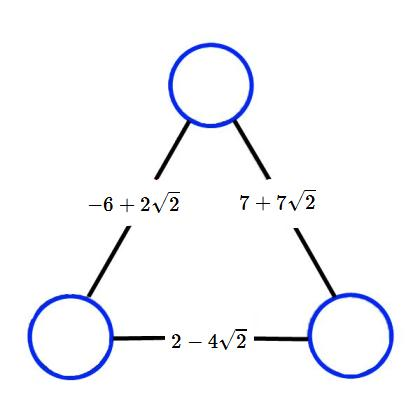#### You may also like### Baby Circle

A small circle fits between two touching circles so that all three circles touch each other and have a common tangent? What is the exact radius of the smallest circle?### Ab Surd Ity

Find the value of sqrt(2+sqrt3)-sqrt(2-sqrt3)and then of cuberoot(2+sqrt5)+cuberoot(2-sqrt5).### Absurdity Again

What is the value of the integers a and b where sqrt(8-4sqrt3) = sqrt a - sqrt b?

# Irrational Arithmagons

##### Age 16 to 18 Challenge Level:

In a multiplication arithmagon, the number on each edge of the arithmagon is the product of the numbers at the adjacent vertices.

You may wish to look at Multiplication Arithmagons and develop a general strategy for working out the numbers at the vertices, given the edge numbers, before tackling the question below.

Can you work out the numbers that belong in the circles to make this multiplication arithmagon correct?If you're not sure where to start, click for a series of hints:

Each circle will be of the form $a+b\sqrt2$ for some values of $a$ and $b$.

In general, a multiplication arithmagon can be solved by multiplying, dividing, and square-rooting.

Dividing an expression by $a+b\sqrt2$ is the same as saying, "What must I multiply $a+b\sqrt2$ by to get that expression?"

To find the square root of an expression such as $12-8\sqrt2$, consider the equation $(x+y\sqrt2)^2=12-8\sqrt2$, expand the brackets, and deduce $x$ and $y$.